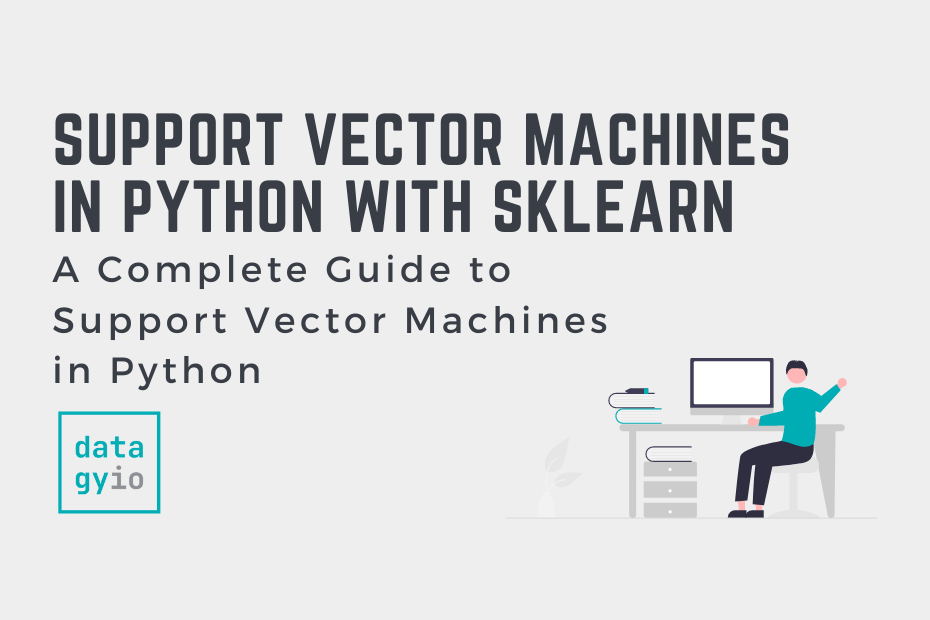# Scikit-Learn## Support Vector Machines (SVM) in Python with Sklearn

In this tutorial, you’ll learn about Support Vector Machines (or SVM) and how they are implemented in Python using Sklearn. The support vector machine algorithm is a supervised machine learning algorithm that is often used for classification problems, though it… Read More »Support Vector Machines (SVM) in Python with Sklearn## One-Hot Encoding in Scikit-Learn with OneHotEncoder

In this tutorial, you’ll learn how to use the OneHotEncoder class in Scikit-Learn to one hot encode your categorical data in sklearn. One-hot encoding is a process by which categorical data (such as nominal data) are converted into numerical features… Read More »One-Hot Encoding in Scikit-Learn with OneHotEncoder## How to Calculate Mean Absolute Error (MAE) in Python

In this tutorial, you’ll learn how to calculate the mean absolute error, or MAE, in Python. The mean absolute error can help measure the accuracy of a given machine learning model. The MAE can be a good complement or alternative to… Read More »How to Calculate Mean Absolute Error (MAE) in Python## K-Nearest Neighbor (KNN) Algorithm in Python

In this tutorial, you’ll learn how all you need to know about the K-Nearest Neighbor algorithm and how it works using Scikit-Learn in Python. The K-Nearest Neighbor algorithm in this tutorial will focus on classification problems, though many of the… Read More »K-Nearest Neighbor (KNN) Algorithm in Python## How to Calculate MAPE in Python

In this tutorial, you’ll learn how to use Python to calculate the MAPE, or the mean absolute percentage error. This error is often used to measure the accuracy of machine learning models. By the end of this tutorial, you’ll have… Read More »How to Calculate MAPE in Python## Hyper-parameter Tuning with GridSearchCV in Sklearn

In this tutorial, you’ll learn how to use GridSearchCV for hyper-parameter tuning in machine learning. In machine learning, you train models on a dataset and select the best performing model. One of the tools available to you in your search… Read More »Hyper-parameter Tuning with GridSearchCV in Sklearn## How to Calculate Mean Squared Error in Python

The mean squared error is a common way to measure the prediction accuracy of a model. In this tutorial, you’ll learn how to calculate the mean squared error in Python. You’ll start off by learning what the mean squared error… Read More »How to Calculate Mean Squared Error in Python## Introduction to Scikit-Learn (sklearn) in Python

In this tutorial, you’ll learn what Scikit-Learn is, how it’s used, and what its basic terminology is. While Scikit-learn is just one of several machine learning libraries available in Python, it is one of the best known. The library provides… Read More »Introduction to Scikit-Learn (sklearn) in Python## Splitting Your Dataset with Scitkit-Learn train_test_split

In this tutorial, you’ll learn how to split your Python dataset using Scikit-Learn’s train_test_split function. You’ll gain a strong understanding of the importance of splitting your data for machine learning to avoid underfitting or overfitting your models. You’ll also learn… Read More »Splitting Your Dataset with Scitkit-Learn train_test_split## Linear Regression in Scikit-Learn (sklearn): An Introduction

In this tutorial, you’ll learn how to learn the fundamentals of linear regression in Scikit-Learn. Throughout this tutorial, you’ll use an insurance dataset to predict the insurance charges that a client will accumulate, based on a number of different factors. You’ll… Read More »Linear Regression in Scikit-Learn (sklearn): An Introduction## Introduction to Random Forests in Scikit-Learn (sklearn)

In this tutorial, you’ll learn what random forests in Scikit-Learn are and how they can be used to classify data. Decision trees can be incredibly helpful and intuitive ways to classify data. However, they can also be prone to overfitting,… Read More »Introduction to Random Forests in Scikit-Learn (sklearn)